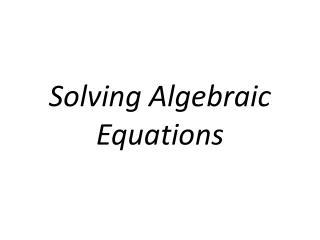DownloadDownload PresentationSolving Algebraic Equations

# Solving Algebraic Equations

Download Presentation## Solving Algebraic Equations

- - - - - - - - - - - - - - - - - - - - - - - - - - - E N D - - - - - - - - - - - - - - - - - - - - - - - - - - -
##### Presentation Transcript

1. Solving Algebraic Equations

2. Equality3 = 33+4 = 3+43+4 = 7For what value of x is: x + 4 = 7 true?Answer: when x=3, then the equation is true.

3. How do I Solve Algebraic Equations? What ever you add, subtract, multiply or divide to one side of the equation, you have to do the same to the other side of the equation! Try to isolate the variable to one side of the equation. Always substitute your answer for the variable to see if the answer and equation are true!

4. Example #1: x+4=7 x+4+(-4) = 7 + (-4) x + 0 = 3 x = 3 Substituting: 3 + 4 = 7 7 = 7 (CHECK!)

5. Example #2: 2x=4 2∙x 4 1∙x = 2 x = 2 = 2 2 Substituting: 2x= 4 2∙2= 4 4 = 4 (CHECK!)

6. PROBLEMS: Solving Algebraic Equations Are you ready… 3,2,1… here we go!

7. Solve the Equation: v – 10 = -9

8. Answer:v - 10 +10 = -9+10v + 0 = 1v = 1

9. Solve the Equation: v – 10 = -3

10. Answer:v – 10 +10 = -3 +10v + 0 = 7v = 7

11. Solve the Equation: x – 3 = 4

12. Answer:x – 3 +3 = 4 +3x + 0 = 7x = 7

13. Note: x – 3 = 4 X = 4 + 3 X = 7

14. Getting back to Solving Equations… 3,2,1… here we go!

15. Solve the Equation: ― = 2 x 5

16. Answer:(5)∙― = (5)∙21 ∙ x = 5∙2x = 5∙2(What do you notice?)x = 10 x5

17. Solve the Equation: 22 = -11k

18. Answer:=-2 = 1k-2 = kk = -2 22 -11 -11k -11

19. Solve the Equation: -13m = -377

21. Solve the Equation: p + 8 = 14.1

23. Solve the Equation: n + 4.7 = -4.7

25. Solve the Equation: n + 3.9 = 0.7

27. Solve the Equation: ― = -7 x 1.2

29. Solve the Equation: -6.3k = -8.19

31. Solve the Equation: 5½ + p = 6

33. Solve the Equation: x – 3 = -5½

35. Solve the Equation: 9⅓ = ⁵⁄₃ ∙n

37. Solve the Equation: ―n = -1― 9 1 10 10

39. Word Problems

40. Solve the word problem: A number increased by 34 is 134.

41. Answer:n + 34 = 134n = 100

42. Solve the word problem: Find the number Seventy-five less than a number is 62.

43. Answer:n - 75 = 62n = 137

44. Solve the word problem: A number increased by 14 is 49.

45. Answer:n + 14 = 49n = 35

46. Solve the word problem: Find the number Twenty-nine more than a number is 52.

47. Answer:n + 29 = 52n = 23

48. Solve the word problem: Find the number One-half of a number is 9.